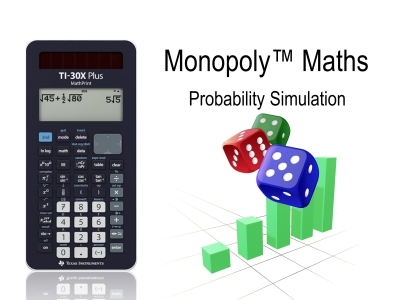# Activities

•• ##### AuthorAust Senior

45 Minutes

## Monopoly Simulation#### Activity Overview

Students review probability as a measure of likely chance to occur, perform simulations of experiments using technology including the identification of factors that could complicate the simulation of real events, create and use a sample space to determine theoretical probabilities and expected values, all through the context of the popular board game Monopoly™.

#### Objectives

This activity has multiple aims, including the ability of students to determine the validity of a digital simulation and provide an appropriate comparison and dialogue comparing practical (simulated) results and theoretical outcomes in the context of a Monopoly game (sum of two dice).

#### Vocabulary

• Empirical data
• Simulation
• Likely - Unlikely
• Sample Space
• Frequency Table

#### About the Lesson

Students use estimate probabilities based on their prior knowledge then simulate them using the calculator. Students compare the simulated results with what they believed and then proceed to produce a sample space to determine the theoretical probabilities. At the end of the activity students are also required to identify the validity of alternative simulation options.